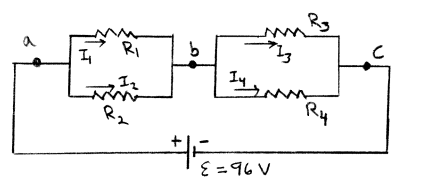Problem: Consider the circuit shown in the sketch. R1 = 3.0 Ω, R2 = 6.0 Ω, R3 = 6.0 Ω. and R4 = 12.0 Ω. What is the current through each resistor?a) I1 =b) I2 = c) I3 = d) I4 =

92% (15 ratings)
Problem Details

Consider the circuit shown in the sketch. R= 3.0 Ω, R= 6.0 Ω, R= 6.0 Ω. and R= 12.0 Ω. What is the current through each resistor?

a) I=

b) I

c) I

d) I=